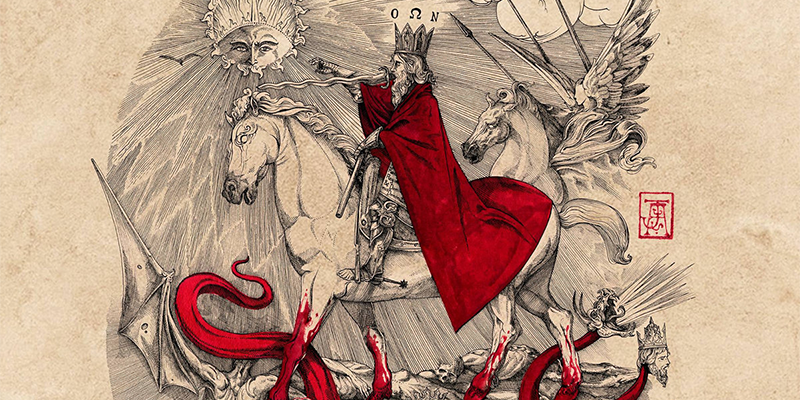《深入理解神经网络 从逻辑回归到CNN》(张觉非)【摘要 书评 试读】- 京东图书item.jd.com1. F 是上下左右翻转后再与 G 对准的。因为卷积公式中 F(s, t) 乘上的是 G(x-s, y-t) 。比如 F(-1, -1) 乘上的是 G(7, 7) ；
2. 如果 F 的所有值之和不等于 1.0，则 C 值有可能不落在 [0, 255] 区间内，那就不是一个合法的图像灰度值。所以如果需要让结果是一幅图像，就得将 F 归一化——令它的所有位置之和等于 1.0 ；
3. 对于 G 边缘上的点，有可能它的周围位置超出了图像边缘。此时可以把图像边缘之外的值当做 0 。或者只计算其周围都不超边缘的点的 C 。这样计算出来的图像就比原图像小一些。在上例中是小了一圈，如果 F 覆盖范围更大，那么小的圈数更多。

+----+----+----+
| -1 |  0 |  1 |
+----+----+----+
| -2 |  0 |  2 |
+----+----+----+
| -1 |  0 |  1 |
+----+----+----+是神经元的输入。a 是神经元的输出。神经元将输入加权求和后再加上偏置值 b ，最后再施加一个函数 f ，即：e 称为均方误差 mse 。全部输出向量和目标输出向量之间的距离（差的模）越小，则 e 越小。e 越小则神经网络的行为与想要的行为越接近。

1500 轮过后，mse 基本就是 0 了。训练完成后网络的权值是：

+-------+--------+--------+
| 1.29  |  0.04  |  -1.31 |
+-------+--------+--------+
| 1.43  |  0.01  |  -1.45 |
+-------+--------+--------+
| 1.34  |  -0.07 |  -1.28 |
+-------+--------+--------+

+----+----+----+
| -1 |  0 |  1 |
+----+----+----+
| -2 |  0 |  2 |
+----+----+----+
| -1 |  0 |  1 |
+----+----+----+precision    recall  f1-score   support

0       0.96      0.98      0.97       252
1       0.99      0.99      0.99       281
2       0.98      0.94      0.96       240
3       0.97      0.95      0.96       258
4       0.96      0.92      0.94       239
5       0.98      0.95      0.97       219
6       0.96      0.99      0.97       273
7       0.97      0.97      0.97       259
8       0.92      0.96      0.94       231
9       0.91      0.97      0.94       248

avg / total       0.96      0.96      0.96      2500


import pandas as pd
from keras.models import Sequential
from keras.layers import Dense, Flatten, Reshape, AveragePooling2D, Convolution2D, Activation
from keras.utils.np_utils import to_categorical
from keras.utils.visualize_util import plot
from sklearn.model_selection import train_test_split
from sklearn.metrics import classification_report, accuracy_score, confusion_matrix
from keras.callbacks import Callback
from keras.optimizers import SGD

class LossHistory(Callback):
def __init__(self):
Callback.__init__(self)
self.losses = []
self.accuracies = []

def on_train_begin(self, logs=None):
pass

def on_batch_end(self, batch, logs=None):
self.losses.append(logs.get('loss'))
self.accuracies.append(logs.get('acc'))

history = LossHistory()

data = data.sample(n=10000, replace=False)
digits = data[data.columns.values[1:]].values
labels = data.label.values

train_digits, test_digits, train_labels, test_labels = train_test_split(digits, labels)

train_labels_one_hot = to_categorical(train_labels)
test_labels_one_hot = to_categorical(test_labels)

model = Sequential()
Convolution2D(nb_filter=32, nb_row=3, nb_col=3, dim_ordering="th", border_mode="same", bias=False, init="uniform"))
Convolution2D(nb_filter=64, nb_row=3, nb_col=3, dim_ordering="th", border_mode="same", bias=False, init="uniform"))

with open("digits_model.json", "w") as f:
f.write(model.to_json())

plot(model, to_file="digits_model.png", show_shapes=True)

opt = SGD(lr=0.01, decay=1e-6, momentum=0.9, nesterov=True)
model.compile(loss="mse", optimizer=opt, metrics=["accuracy"])

model.fit(train_digits, train_labels_one_hot, batch_size=32, nb_epoch=10, callbacks=[history])

model.save_weights("digits_model_weights.hdf5")

predict_labels = model.predict_classes(test_digits)

print(classification_report(test_labels, predict_labels))
print(accuracy_score(test_labels, predict_labels))
print(confusion_matrix(test_labels, predict_labels))



from keras.models import Sequential
from keras.layers import Convolution2D
from keras.callbacks import Callback
from PIL import Image
import numpy as np
from scipy.ndimage.filters import convolve

class LossHistory(Callback):
def __init__(self):
Callback.__init__(self)
self.losses = []

def on_train_begin(self, logs=None):
pass

def on_batch_end(self, batch, logs=None):
self.losses.append(logs.get('loss'))

lena = np.array(Image.open("lena.png").convert("L"))
lena_sobel = np.zeros(lena.shape)

# sobel 算子。
sobel = np.array([
[-1, 0, 1],
[-2, 0, 2],
[-1, 0, 1]
])

# 计算卷积：用 sobel 算子滤波。结果保存在 lena_sobel 中。
convolve(input=lena, output=lena_sobel, weights=sobel, mode="constant", cval=1.0)

# 将像素值调整到 [0，255] 区间并保存 sobel 算子滤波后的 lena 图。
lena_tmp = np.uint8((lena_sobel - lena_sobel.min()) * 255 / (lena_sobel.max() - lena_sobel.min()))
Image.fromarray(lena_tmp).save("lena_sobel.png")

# 将原始 lena 图和 sobel 滤波 lena 图转换成 (1, 1, width, height) 尺寸。第一个 1 表示训练集只有一个样本。第二个 1 表示样本只有一个 channel 。
X = lena.reshape((1, 1) + lena.shape)
Y = lena_sobel.reshape((1, 1) + lena_sobel.shape)

# 建一个神经网络模型。
model = Sequential()

# 只添加一个卷积层。卷积层只有一个滤波器。滤波器尺寸 3x3 。输入维度顺序是 "th" 表示 (channel, width, height) 。输入尺寸是 (channel, width, height) 。不要偏执置。
Convolution2D(nb_filter=1, nb_row=3, nb_col=3, dim_ordering="th", input_shape=X.shape[1:], border_mode="same",
bias=False, init="uniform"))

# 代价函数取 mse 。优化算法取 rmsprop 。
model.compile(loss="mse", optimizer="rmsprop", metrics=["accuracy"])

history = LossHistory()

# 训练 10 轮，每轮保存一下当前网络输出图像。
for i in np.arange(0, 10):
lena_tmp = model.predict(X).reshape(lena.shape)
lena_tmp = np.uint8((lena_tmp - lena_tmp.min()) * 255 / (lena_tmp.max() - lena_tmp.min()))
Image.fromarray(lena_tmp).save("lena_sobel_stage_{:d}.png".format(i))
print("lena_sobel_stage_{:d}.png saved".format(i))

model.fit(X, Y, batch_size=1, nb_epoch=200, verbose=1, callbacks=[history])
print("Epoch {:d}".format(i + 1))

lena_tmp = model.predict(X).reshape(lena.shape)
lena_tmp = np.uint8((lena_tmp - lena_tmp.min()) * 255 / (lena_tmp.max() - lena_tmp.min()))
Image.fromarray(lena_tmp).save("lena_sobel_stage_final.png")